Anzeige

# Introduction to Reinforcement Learning for Molecular Design

6. Dec 2018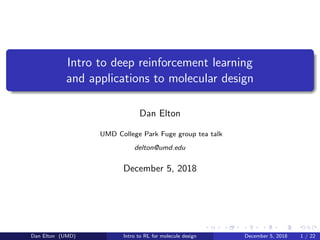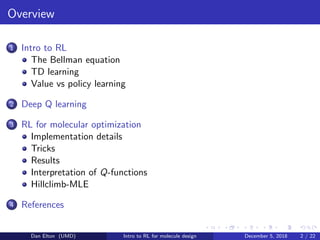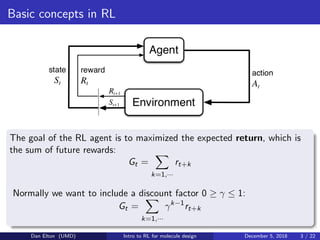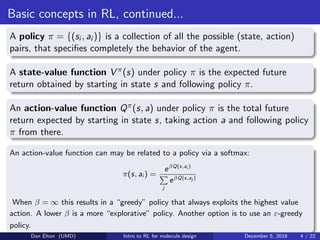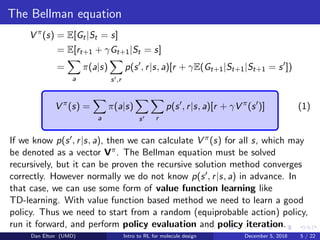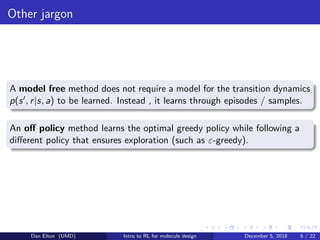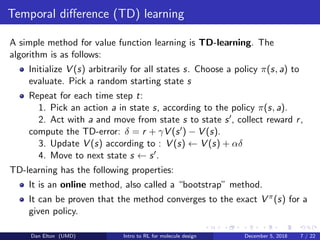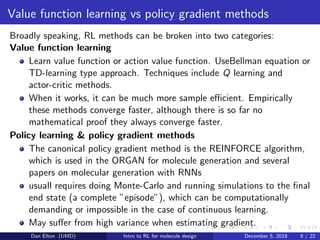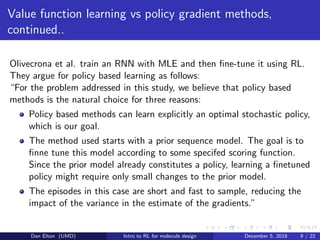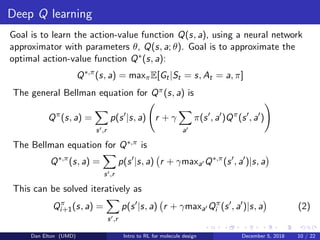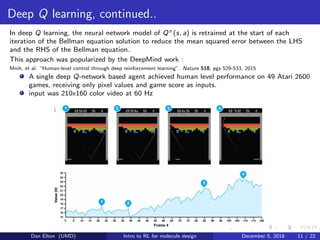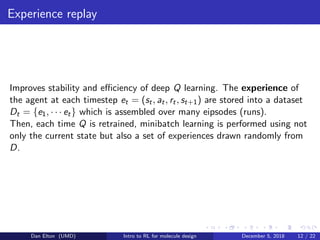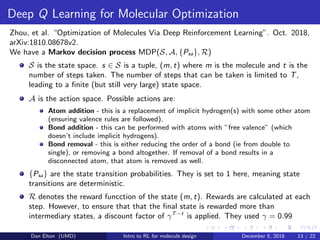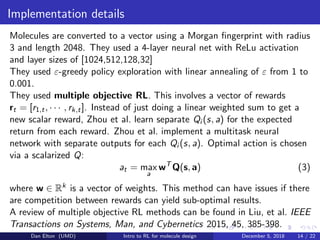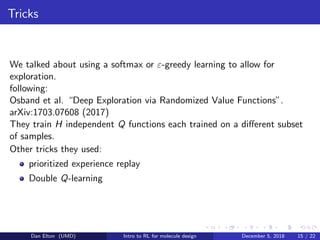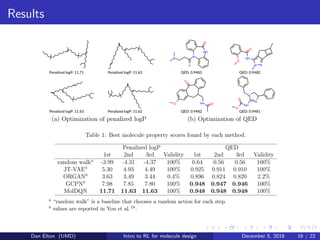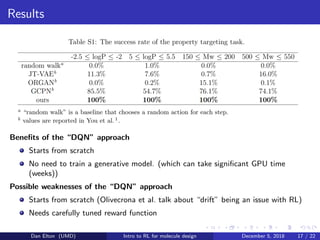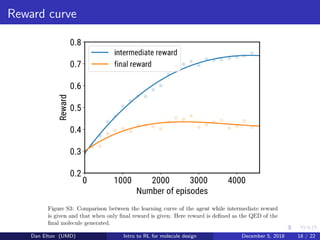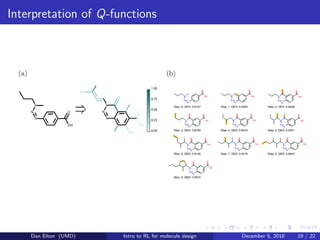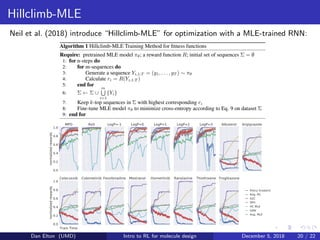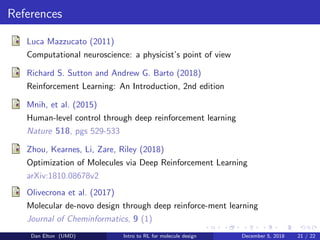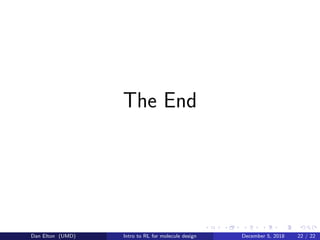1 von 22
Anzeige

### Introduction to Reinforcement Learning for Molecular Design

1. Intro to deep reinforcement learning and applications to molecular design Dan Elton UMD College Park Fuge group tea talk delton@umd.edu December 5, 2018 Dan Elton (UMD) Intro to RL for molecule design December 5, 2018 1 / 22
2. Overview 1 Intro to RL The Bellman equation TD learning Value vs policy learning 2 Deep Q learning 3 RL for molecular optimization Implementation details Tricks Results Interpretation of Q-functions Hillclimb-MLE 4 References Dan Elton (UMD) Intro to RL for molecule design December 5, 2018 2 / 22
3. Basic concepts in RL The goal of the RL agent is to maximized the expected return, which is the sum of future rewards: Gt = k=1,··· rt+k Normally we want to include a discount factor 0 ≥ γ ≤ 1: Gt = k=1,··· γk−1 rt+k Dan Elton (UMD) Intro to RL for molecule design December 5, 2018 3 / 22
4. Basic concepts in RL, continued... A policy π = {(si , ai )} is a collection of all the possible (state, action) pairs, that speciﬁes completely the behavior of the agent. A state-value function V π(s) under policy π is the expected future return obtained by starting in state s and following policy π. An action-value function Qπ(s, a) under policy π is the total future return expected by starting in state s, taking action a and following policy π from there. An action-value function can may be related to a policy via a softmax: π(s, ai ) = eβQ(s,ai ) j eβQ(s,aj ) When β = ∞ this results in a “greedy” policy that always exploits the highest value action. A lower β is a more “explorative” policy. Another option is to use an ε-greedy policy. Dan Elton (UMD) Intro to RL for molecule design December 5, 2018 4 / 22
5. The Bellman equation V π (s) = E[Gt|St = s] = E[rt+1 + γGt+1|St = s] = a π(a|s) s ,r p(s , r|s, a)[r + γE(Gt+1|St+1|St+1 = s ]) V π (s) = a π(a|s) s r p(s , r|s, a)[r + γV π (s )] (1) If we know p(s , r|s, a), then we can calculate V π(s) for all s, which may be denoted as a vector Vπ. The Bellman equation must be solved recursively, but it can be proven the recursive solution method converges correctly. However normally we do not know p(s , r|s, a) in advance. In that case, we can use some form of value function learning like TD-learning. With value function based method we need to learn a good policy. Thus we need to start from a random (equiprobable action) policy, run it forward, and perform policy evaluation and policy iteration. Dan Elton (UMD) Intro to RL for molecule design December 5, 2018 5 / 22
6. Other jargon A model free method does not require a model for the transition dynamics p(s , r|s, a) to be learned. Instead , it learns through episodes / samples. An oﬀ policy method learns the optimal greedy policy while following a diﬀerent policy that ensures exploration (such as ε-greedy). Dan Elton (UMD) Intro to RL for molecule design December 5, 2018 6 / 22
7. Temporal diﬀerence (TD) learning A simple method for value function learning is TD-learning. The algorithm is as follows: Initialize V (s) arbitrarily for all states s. Choose a policy π(s, a) to evaluate. Pick a random starting state s Repeat for each time step t: 1. Pick an action a in state s, according to the policy π(s, a). 2. Act with a and move from state s to state s , collect reward r, compute the TD-error: δ = r + γV (s ) − V (s). 3. Update V (s) according to : V (s) ← V (s) + αδ 4. Move to next state s ← s . TD-learning has the following properties: It is an online method, also called a “bootstrap” method. It can be proven that the method converges to the exact V π(s) for a given policy. Dan Elton (UMD) Intro to RL for molecule design December 5, 2018 7 / 22
8. Value function learning vs policy gradient methods Broadly speaking, RL methods can be broken into two categories: Value function learning Learn value function or action value function. UseBellman equation or TD-learning type approach. Techniques include Q learning and actor-critic methods. When it works, it can be much more sample eﬃcient. Empirically these methods converge faster, although there is so far no mathematical proof they always converge faster. Policy learning & policy gradient methods The canonical policy gradient method is the REINFORCE algorithm, which is used in the ORGAN for molecule generation and several papers on molecular generation with RNNs usuall requires doing Monte-Carlo and running simulations to the ﬁnal end state (a complete ”episode”), which can be computationally demanding or impossible in the case of continuous learning. May suﬀer from high variance when estimating gradient. Dan Elton (UMD) Intro to RL for molecule design December 5, 2018 8 / 22
9. Value function learning vs policy gradient methods, continued.. Olivecrona et al. train an RNN with MLE and then ﬁne-tune it using RL. They argue for policy based learning as follows: “For the problem addressed in this study, we believe that policy based methods is the natural choice for three reasons: Policy based methods can learn explicitly an optimal stochastic policy, which is our goal. The method used starts with a prior sequence model. The goal is to ﬁnne tune this model according to some specifed scoring function. Since the prior model already constitutes a policy, learning a ﬁnetuned policy might require only small changes to the prior model. The episodes in this case are short and fast to sample, reducing the impact of the variance in the estimate of the gradients.” Dan Elton (UMD) Intro to RL for molecule design December 5, 2018 9 / 22
10. Deep Q learning Goal is to learn the action-value function Q(s, a), using a neural network approximator with parameters θ, Q(s, a; θ). Goal is to approximate the optimal action-value function Q∗(s, a): Q∗,π (s, a) = maxπE[Gt|St = s, At = a, π] The general Bellman equation for Qπ(s, a) is Qπ (s, a) = s ,r p(s |s, a) r + γ a π(s , a )Qπ (s , a ) The Bellman equation for Q∗,π is Q∗,π (s, a) = s ,r p(s |s, a) r + γmaxa Q∗,π (s , a )|s, a This can be solved iteratively as Qπ i+1(s, a) = s ,r p(s |s, a) r + γmaxa Qπ i (s , a )|s, a (2) Dan Elton (UMD) Intro to RL for molecule design December 5, 2018 10 / 22
11. Deep Q learning, continued.. In deep Q learning, the neural network model of Qπ(s, a) is retrained at the start of each iteration of the Bellman equation solution to reduce the mean squared error between the LHS and the RHS of the Bellman equation. This approach was popularized by the DeepMind work : Mnih, et al. “Human-level control through deep reinforcement learning”. Nature 518, pgs 529-533, 2015 A single deep Q-network based agent achieved human level performance on 49 Atari 2600 games, receiving only pixel values and game score as inputs. input was 210x160 color video at 60 Hz Dan Elton (UMD) Intro to RL for molecule design December 5, 2018 11 / 22
12. Experience replay Improves stability and eﬃciency of deep Q learning. The experience of the agent at each timestep et = (st, at, rt, st+1) are stored into a dataset Dt = {e1, · · · et} which is assembled over many eipsodes (runs). Then, each time Q is retrained, minibatch learning is performed using not only the current state but also a set of experiences drawn randomly from D. Dan Elton (UMD) Intro to RL for molecule design December 5, 2018 12 / 22
13. Deep Q Learning for Molecular Optimization Zhou, et al. “Optimization of Molecules Via Deep Reinforcement Learning”. Oct. 2018, arXiv:1810.08678v2. We have a Markov decision process MDP(S, A, {Psa}, R) S is the state space. s ∈ S is a tuple, (m, t) where m is the molecule and t is the number of steps taken. The number of steps that can be taken is limited to T, leading to a ﬁnite (but still very large) state space. A is the action space. Possible actions are: Atom addition - this is a replacement of implicit hydrogen(s) with some other atom (ensuring valence rules are followed). Bond addition - this can be performed with atoms with ”free valence” (which doesn’t include implicit hydrogens). Bond removal - this is either reducing the order of a bond (ie from double to single), or removing a bond altogether. If removal of a bond results in a disconnected atom, that atom is removed as well. {Psa} are the state transition probabilities. They is set to 1 here, meaning state transitions are deterministic. R denotes the reward funcction of the state (m, t). Rewards are calculated at each step. However, to ensure that that the ﬁnal state is rewarded more than intermediary states, a discount factor of γT−t is applied. They used γ = 0.99 Dan Elton (UMD) Intro to RL for molecule design December 5, 2018 13 / 22
14. Implementation details Molecules are converted to a vector using a Morgan ﬁngerprint with radius 3 and length 2048. They used a 4-layer neural net with ReLu activation and layer sizes of [1024,512,128,32] They used ε-greedy policy exploration with linear annealing of ε from 1 to 0.001. They used multiple objective RL. This involves a vector of rewards rt = [r1,t, · · · , rk,t]. Instead of just doing a linear weighted sum to get a new scalar reward, Zhou et al. learn separate Qi (s, a) for the expected return from each reward. Zhou et al. implement a multitask neural network with separate outputs for each Qi (s, a). Optimal action is chosen via a scalarized Q: at = max a wT Q(s, a) (3) where w ∈ Rk is a vector of weights. This method can have issues if there are competition between rewards can yield sub-optimal results. A review of multiple objective RL methods can be found in Liu, et al. IEEE Transactions on Systems, Man, and Cybernetics 2015, 45, 385-398. Dan Elton (UMD) Intro to RL for molecule design December 5, 2018 14 / 22
15. Tricks We talked about using a softmax or ε-greedy learning to allow for exploration. following: Osband et al. “Deep Exploration via Randomized Value Functions”. arXiv:1703.07608 (2017) They train H independent Q functions each trained on a diﬀerent subset of samples. Other tricks they used: prioritized experience replay Double Q-learning Dan Elton (UMD) Intro to RL for molecule design December 5, 2018 15 / 22
16. Results Dan Elton (UMD) Intro to RL for molecule design December 5, 2018 16 / 22
17. Results Beneﬁts of the “DQN” approach Starts from scratch No need to train a generative model. (which can take signiﬁcant GPU time (weeks)) Possible weaknesses of the “DQN” approach Starts from scratch (Olivecrona et al. talk about “drift” being an issue with RL) Needs carefully tuned reward function Dan Elton (UMD) Intro to RL for molecule design December 5, 2018 17 / 22
18. Reward curve Dan Elton (UMD) Intro to RL for molecule design December 5, 2018 18 / 22
19. Interpretation of Q-functions Dan Elton (UMD) Intro to RL for molecule design December 5, 2018 19 / 22
20. Hillclimb-MLE Neil et al. (2018) introduce “Hillclimb-MLE” for optimization with a MLE-trained RNN: Dan Elton (UMD) Intro to RL for molecule design December 5, 2018 20 / 22
21. References Luca Mazzucato (2011) Computational neuroscience: a physicist’s point of view Richard S. Sutton and Andrew G. Barto (2018) Reinforcement Learning: An Introduction, 2nd edition Mnih, et al. (2015) Human-level control through deep reinforcement learning Nature 518, pgs 529-533 Zhou, Kearnes, Li, Zare, Riley (2018) Optimization of Molecules via Deep Reinforcement Learning arXiv:1810.08678v2 Olivecrona et al. (2017) Molecular de-novo design through deep reinforce-ment learning Journal of Cheminformatics, 9 (1) Dan Elton (UMD) Intro to RL for molecule design December 5, 2018 21 / 22
22. The End Dan Elton (UMD) Intro to RL for molecule design December 5, 2018 22 / 22
Anzeige